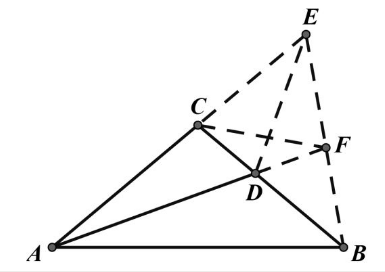【答案】 简证: 作 $B E$ 使得 $\angle A B E=80^{\circ}$ 交直线 $A C$ 于 $E, A D$ 延长线与 $B E$ 交于点 $F$ 则 $B C$ 是 $\angle A B E$ 的平分线, $\angle C A B=40^{\circ}$
\begin{aligned} & \angle A E B=60^{\circ} \\ & \angle C D F=120^{\circ}, C 、 D 、 F 、 E \text { 四点共圆 } \\ & \angle D F C=\angle D E C=\angle D E F=\angle D C F \\ & C D=D F, A D+C D=A F=A B \text { 。 } \end{aligned}